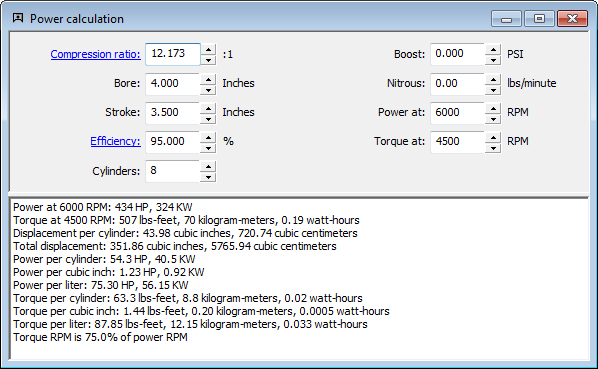## Power Calculation

The power calculation will estimate the horsepower and torque produced by any spark-ignition engine except rotary engines. Turbocharger or supercharger boost and/or nitrous oxide injection are supported. This calculation does not give you the detailed information available from a full engine simulation (it calculates peak power and torque rather than a power curve), but it is surprisingly accurate and is quick and easy to use, requiring only a minimal amount of information that is readily available for most any engine.This calculation determines horsepower and torque based on compression ratio, bore, stroke, efficiency, number of cylinders, boost pressure (if any), nitrous flow rate (if any). Power and torque are calculated for the specified engine speeds.

Also calculated are total displacement, displacement per cylinder, power per cylinder, power per cubic inch, power per liter, torque per cylinder, torque per cubic inch, torque per liter, and ratio of torque RPM to power RPM.

A performance-based power calculation and a performance-based torque calculation is also provided.